## How to quickly calculate the overtime and payment in Excel?

For instance, there is a table recording a worker’s work on and work off time, and you want to calculate the overtime hour and the payment as below screenshot shown, how can you quickly solve this task in Excel?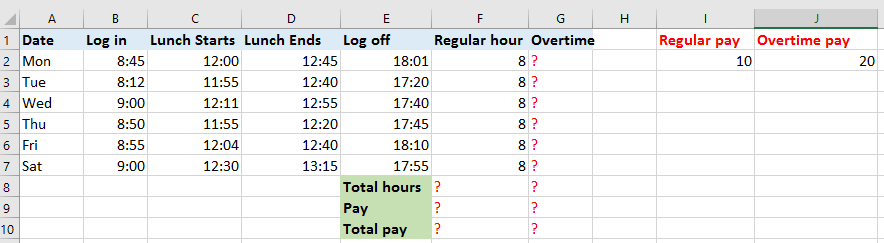Calculate overtime and payment

####Calculate overtime and payment

To calculate the overtime hour and payment, you can do as below:

1. Apply a formula to display the regular working hour first. In cell F1, enter this formula =IF((((C2-B2)+(E2-D2))*24)>8,8,((C2-B2)+(E2-D2))*24), and drag auto fill handle down to display the regular working hour of each day. See screenshot: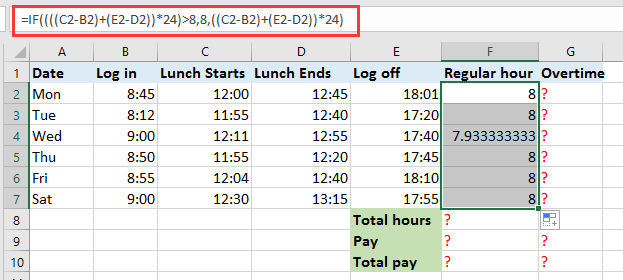2. In cell G2, which will display the overtime, enter this formula =IF(((C2-B2)+(E2-D2))*24>8, ((C2-B2)+(E2-D2))*24-8,0), drag AutoFill handle down to apply this formula to the cells, now the overtime of each day has been calculated. See screenshot: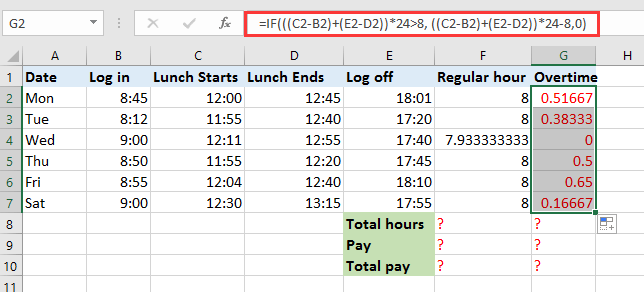In above formulas, C2 and D2 are the time that lunch starts and ends, and B2 and E2 are the time that log in and log off, 8 is the regular working hour each day, you can change these references as you need.

You can calculate the total working hours and payments.

3. In cell F8, type this formula =SUM(F2:F7) and drag to right to fill this formula to G8, now the total regular hours and overtime hours have been gotten.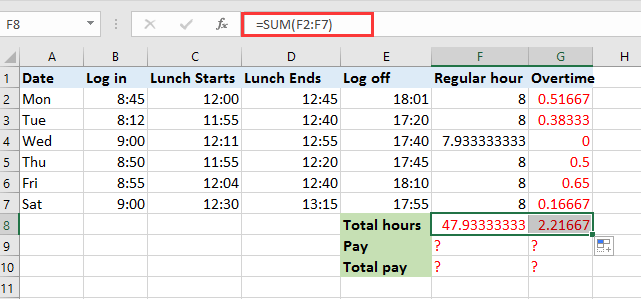4. In the cells which you want to calculate the payment for regular hours and overtime, enter the formula =F8*I2 and formula =G8*J2 separately, see screenshots:

In the formula, F8 and G8 are the cells containing total regular hours and total overtime, I2 and J2 contain the payment per hour.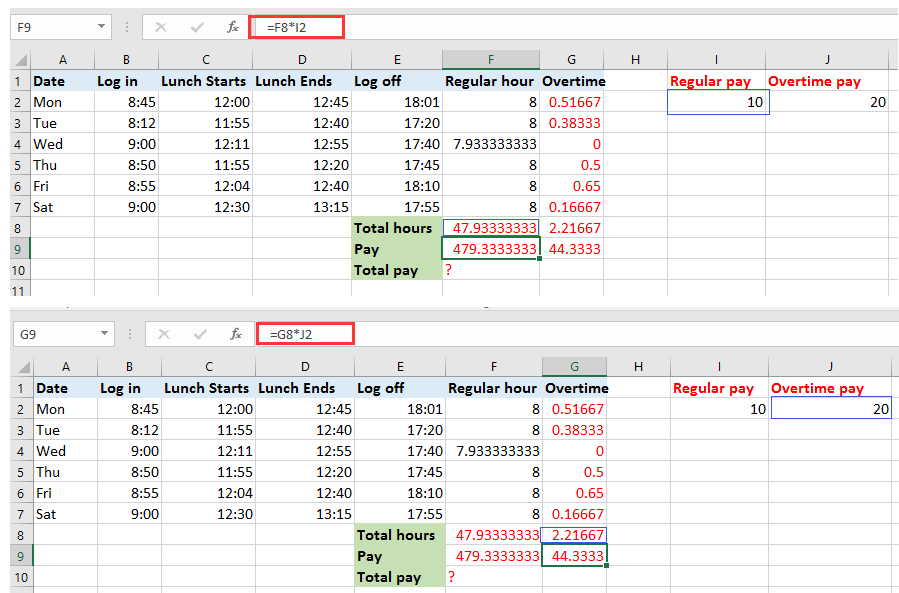You can apply =F9+G9 to calculate the total payments which include regular payments and overtime payment.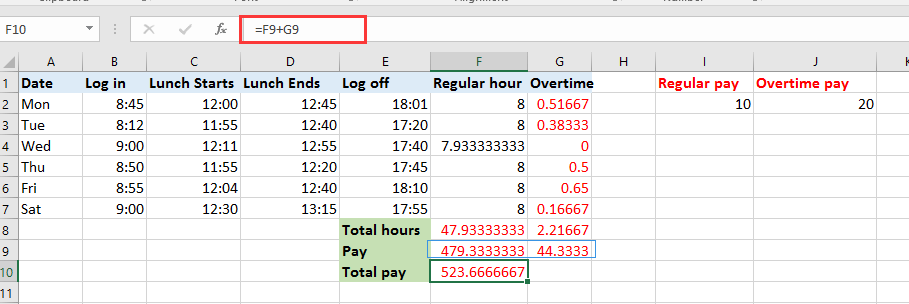#### Easily add days/years/month/hours/minutes/seconds to a datetime in Excel

Supposing you have a date time format data in a cell, and now you need to add a number of days, years, months, hours, minutes, or seconds to this date. Normally, using formula is the first method for all Excel users, but it’s hard to remember all formulas. With Kutools for Excel’s Date & Time Helper utility, you can easily add days, years, months, or hours, minutes or seconds to a date time, moreover, you can caculate the date difference, or the age based on a given birthday without remembering the formula at all. Click for full-featured free trial in 30 days!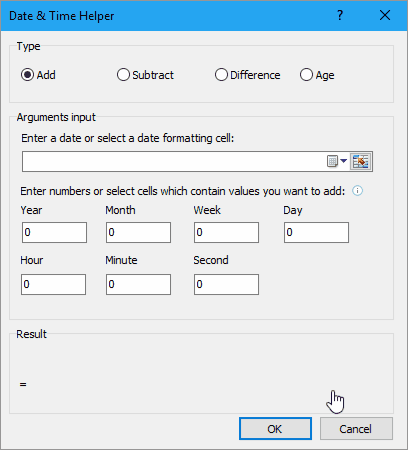### Best Office Productivity Tools

Supercharge Your Spreadsheets： Experience Efficiency Like Never Before with Kutools for Excel

 Popular Features: Find/Highlight/Identify Duplicates   |  Delete Blank Rows   |  Combine Columns or Cells without Losing Data   |   Round without Formula ... Super Lookup: Multiple Criteria VLookup  |   Multiple Value VLookup  |   VLookup Across Multiple Sheets   |   Fuzzy Lookup .... Advanced Drop-down List: Quickly Create Drop Down List   |  Dependent Drop Down List   |  Multi-select Drop Down List .... Column Manager: Add a Specific Number of Columns   |   Move Columns   |   Unhide Columns   |   Compare Columns to Select Same & Different Cells ... Featured Features: Grid Focus   |  Design View   |   Big Formula Bar   |  Workbook & Sheet Manager   |  Resource Library (Auto Text)   |  Date Picker   |  Combine Worksheets   |  Encrypt/Decrypt Cells   |  Send Emails by List   |  Super Filter   |   Special Filter (filter bold/italic/strikethrough...) ... Top 15 Toolset:  12 Text Tools (Add Text, Remove Characters, ...)   |   50+ Chart Types (Gantt Chart, ...)   |   40+ Practical Formulas (Calculate age based on birthday, ...)   |   19 Insertion Tools (Insert QR Code, Insert Picture from Path, ...)   |   12 Conversion Tools (Numbers to Words, Currency Conversion, ...)   |   7 Merge & Split Tools (Advanced Combine Rows, Split Cells, ...)   |   Many More...

Kutools for Excel boasts over 300 features, ensuring that what you need is just a click away...

Supports Office/Excel 2007-2021 & newer, including 365   |   Available in 44 languages   |   Enjoy a full-featured 30-day free trial.#### Office Tab Brings Tabbed interface to Office, and Make Your Work Much Easier

• Enable tabbed editing and reading in Word, Excel, PowerPoint, Publisher, Access, Visio and Project.
• Open and create multiple documents in new tabs of the same window, rather than in new windows.
• Increases your productivity by 50%, and reduces hundreds of mouse clicks for you every day!No ratings yet. Be the first to rate!
This comment was minimized by the moderator on the site
=IF(F13<=TIME(10,0,0), IF(C13>F13-TIME(8,48,0)>=TIME(1,0,0),C13-TIME(8,48,0),0), IF(C13>F13-TIME(8,48,0)>=TIME(1,0,0),F13-TIME(8,48,0),0))
The formula works perfectly, except when I insert formula in H13 it shows ####### instead of 00:00
Dates and times that are negative or too large show as #######
Only after I enter times from C13 to E13, it shows time in H13
So, when I insert the formula in column H downwards for each day of the month, it shows #######
There are days when I’m not working, it show ####### and not 00:00
At the bottom in Total =SUM(H13:H44) it shows ####### and no time for the days I worked
This comment was minimized by the moderator on the site
How can I getting excel to calculate overtime in a template where the pay periods are first to the 15 and 16 through 31 st
This comment was minimized by the moderator on the site
hey so i have a timesheet for work i need it to calculate total hours, overtime 1 and overtime 2
where hours from 07:00-15:00 is normal hours so no overtime counted
hours from 15:00-17:00 is counted in overtime 1
and all other hours is counted in overtime 2
like so
if A1(start time)=07:00 B1(end time)=19:00 C1(total hours)=12 D1(overtime1)=2 E1(overtime2)=2
or A1(start time)=04:00 B1(end time)=16:00 C1(total hours)=12 D1(overtime1)=1 E1(overtime2)=3
This comment was minimized by the moderator on the site
This was awesome should add the Double time calculations also. Thank you
There are no comments posted here yet